Calculus 3 : Vectors and Vector Operations

Example Questions

1 2 68 69 70 71 72 73 74 76 Next →

Example Question #751 : Vectors And Vector Operations

Find the determinant of the 3x3 matrix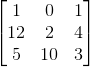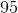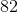Explanation:

To find the determinant of a 3x3 matrix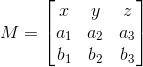, you use the formula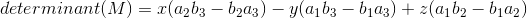Using the matrix from the problem statement, we get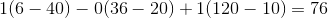Example Question #752 : Vectors And Vector Operations

Find the determinant of the matrix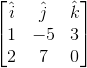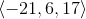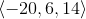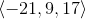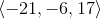Explanation:

To find the determinant of a 3x3 matrix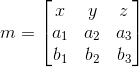, we use the formula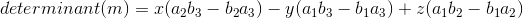Using the matrix from the problem statement, we get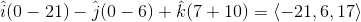Example Question #753 : Vectors And Vector Operations

Calculate the determinant of Matrix.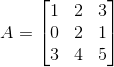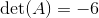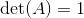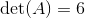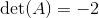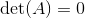Explanation:

In order to find the determinant of, we first need to copy down the first two columns into columns 4 and 5.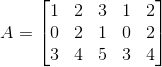The next step is to multiply the down diagonals.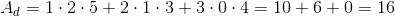The next step is to multiply the up diagonals.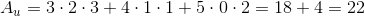The last step is to substract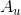from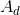.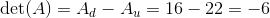Example Question #754 : Vectors And Vector Operations

Calculate the determinant of Matrix.Explanation:

In order to find the determinant of, we first need to copy down the first two columns into columns 4 and 5.The next step is to multiply the down diagonals.The next step is to multiply the up diagonals.The last step is to substractfrom.1 2 68 69 70 71 72 73 74 76 Next →